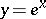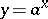# Exponential function, real

(diff) ← Older revision | Latest revision (diff) | Newer revision → (diff)
The function, also denoted by. Sometimes the functionfor any baseis also called an exponential function.
See also Exponential function; Exponent;(number). The inverse of the exponential function is the logarithmic function (cf. also Logarithm of a number). The value of the exponential function at a point is also called the antilogarithm of this point.